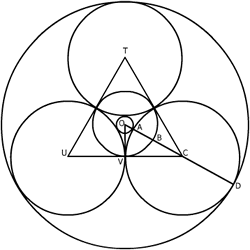#### You may also like### Baby Circle

A small circle fits between two touching circles so that all three circles touch each other and have a common tangent? What is the exact radius of the smallest circle?### Kissing

Two perpendicular lines are tangential to two identical circles that touch. What is the largest circle that can be placed in between the two lines and the two circles and how would you construct it?### Logosquares

Ten squares form regular rings either with adjacent or opposite vertices touching. Calculate the inner and outer radii of the rings that surround the squares.

# Circles in Circles

##### Age 16 to 18 Challenge Level:

This solution together with the diagram was sent in by Derek Wan, age 17 of Sha Tin College, Hong KongTo find $OA=r_1$, $OB=r_2$ and $OD=r_3$ we must examine the diagram and make appropriate considerations. By dropping a perpendicular from $O$ to $V$, the midpoint of $UC$, as the radius is perpendicular to the tangent, we can find $OA = r_1$. Since triangle $TCU$ is equilateral $\angle TCU=60^o$ and $OC$ bisects $\angle TCU$ so $\angle OCV = 30^o$.

Since $OC=OA+AC$ $${CV\over OA+AC}={\sqrt 3 \over 2}$$ As $AC=CV=1$ and $OA=r_1$, $${1\over r_1+1} = {\sqrt 3 \over 2}$$ $$r_1+1 = {2\over \sqrt 3}$$ $$r_1 = {2\over \sqrt 3}-1$$ With $r_1$ we can find $r_2$ and $r_3$. Since $OV=r_2$, $CV=1$ and $\angle OCV=30^0$, $$r_2={1\over \sqrt 3}.$$ Since $OD=OA+AC+CD$, $$r_3={2\over \sqrt 3} - 1 + 1 + 1 = {2\over \sqrt 3} + 1.$$ Now $$r_1r_2 = \left({2\over \sqrt 3 }- 1\right )\left({2\over \sqrt 3} + 1\right )$$ $$\quad = {4\over 3} + {2\over \sqrt 3} - {2\over \sqrt 3} - 1$$ $$\quad = {1\over 3}$$ $$\quad = \left({1\over \sqrt 3}\right)^2$$ $$\quad = r_2^2$$